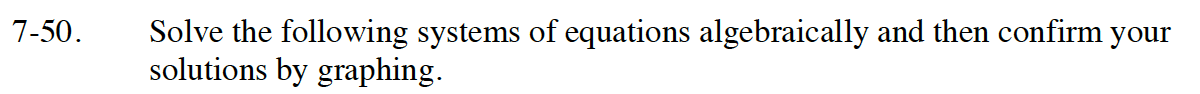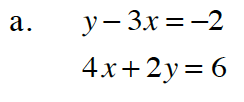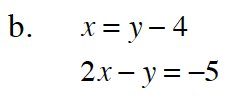Home > CC4 > Chapter 7 > Lesson 7.1.4 > Problem7-50

7-50.
1. Solve the following systems of equations algebraically and then confirm your solutions by graphing. Homework Help ✎

2.  a. y − 3x = −2 4x + 2y = 6 b. x = y − 4 2x − y = −5Read the Math Notes box in Lesson 6.2.3 on the Substitution Method.(1,1)Substitute x = y − 4 in the second equation.